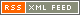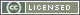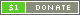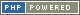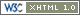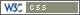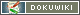# Boolean

A Boolean expresses a "truth" value, and can therefore be `true` or `false`. `true` and `false` are Constants, so can be used in expressions.

For example:

```{ a = true }
{ b = false }

{ if a }
...
{ endif }

{ if a == true }
...
{ endif }

{ if a === true }
...
{ endif }```

Note that in the example above, each `If` statement checks for the "truthfulness" of variable `a`, but the final statement (`if a === true`) also checks that `a` is explicitly boolean. That is because non-explicit statements perform a conversion to Boolean before making the comparison.

### Converting to Boolean

To explicitly convert a value to Boolean, the Boolean typecast operator - `?` - should be used. Some operators, such as the boolean logic NOT operator - `!` or `not` - also explictly convert a value to Boolean.

When converting to Boolean, the following values are considered to be `false`:

• A Boolean with value `false`
• A Number with value zero (`0` or `0.0`)
• An empty String - `""`
• A String containing a single zero - `"0"`
• An empty Array (with no elements)

Every other value is considered to be `true`.

The number `-1` is considered to be `true`, just like any other non-zero number.